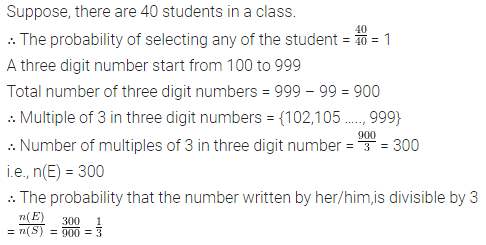# Activity : Ask all the students in your class to write a 3-digit number

Activity : Ask all the students in your class to write a 3-digit number. Choose any student from the room at random. What is the probability that the number written by her/him is divisible by 3? Remember that a number is divisible by 3, if the sum of its digit is divisible by 3.